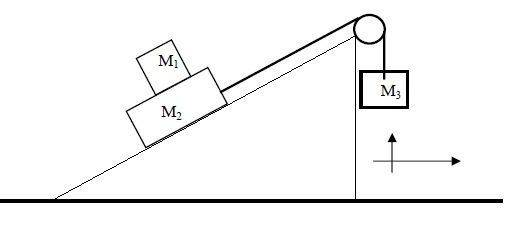# Newton Laws/Dynamics: 3 Masses on an incline

Mu1 is the friction coefficient , I'm sure I did it wrong because I got the acceleration isn't dependent on it, and it doesn't make sense.
disregard the axis in the drawing - it's for another question.

## Homework Statement

Find an expression for the acceleration of the system when there is no relative movement between M1 and M2
(same acceleration and velocity?)ΣF =ma

## The Attempt at a Solution

View attachment 1.doc

If there is no relative movement between M1 and M2 then one can take (M1 + M2) as one body. The coefficient of friction is between the block and the inclined plane. Right?

forgot to mention: there's no friction between the incline and the mass 2 only between mass 1 and 2.
I don't think you can look at it as one body mass 1 can slip from mass 2 the friction is the only think that can make them move together (the only force that resist the earth pull on mass1).

I don't know what the answer is. that's what I'm trying to find out!

It was given that there is no relative movement between M1 and M2. Of course there is friction between these two masses. But if these masses are considered as one body then this friction force will be an internal force and can be left out of our consideration.

Maybe you are right, then it's reasonable why I haven't got Mu in the answer :/
I don't have the final answer so I'm trying to find out if I'm right... these questions have grades.

My line of thinking is that if Mu will be a large number than m1 could go up along with m2 even if m3 is heavy (and thus the force pulling both of the masses up is greater) but if it would be very little m1 won't "keep up" with m2(if it's going up) .
and so the acceleration has to be dependent on Mu - though I could be wrong.

But thanks, it's really kind of you to help :)

It all depends on whether or not it is 'given' that there is no relative motion between M1 and M2.